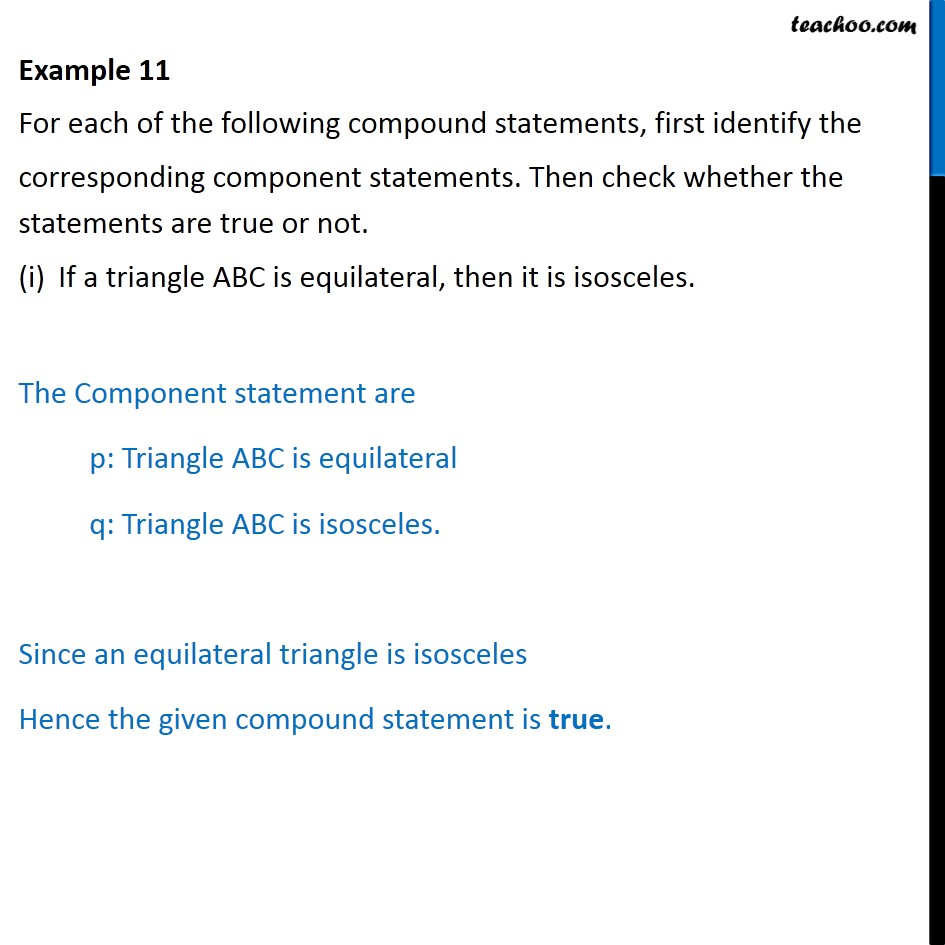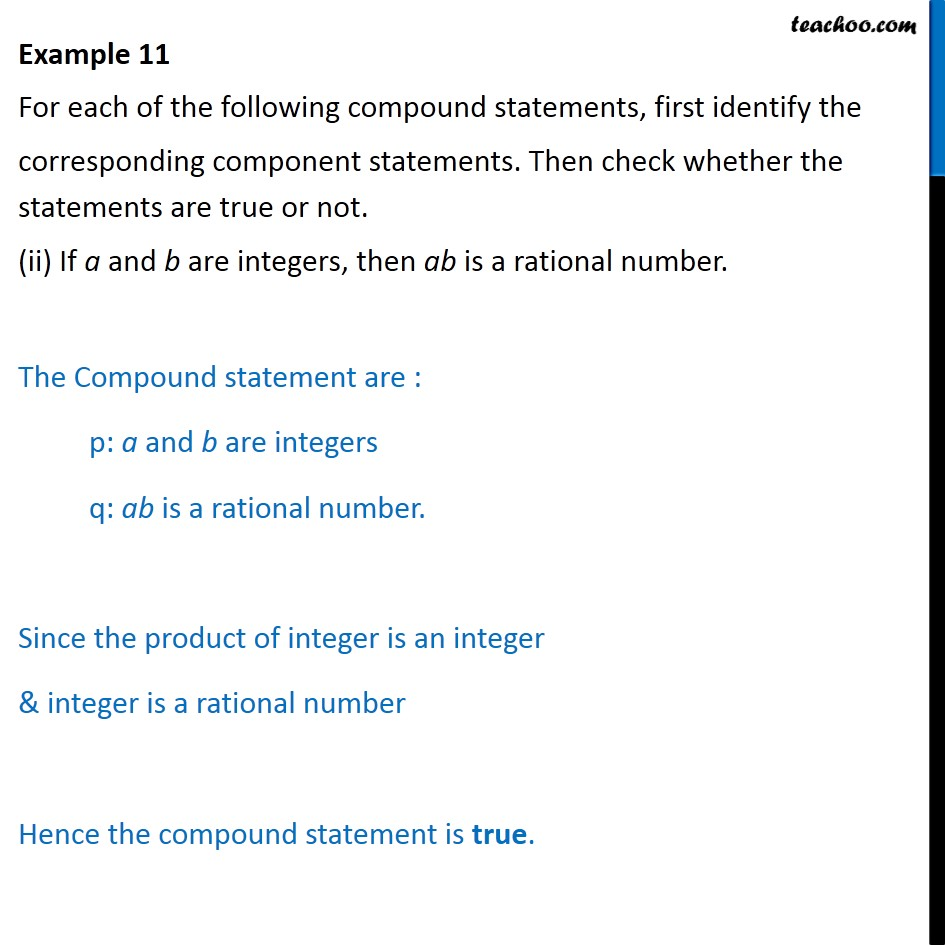Subscribe to our Youtube Channel - https://you.tube/teachoo

1. Chapter 14 Class 11 Mathematical Reasoning
2. Serial order wise
3. Examples

Transcript

Example 11 For each of the following compound statements, first identify the corresponding component statements. Then check whether the statements are true or not. If a triangle ABC is equilateral, then it is isosceles. The Component statement are p: Triangle ABC is equilateral q: Triangle ABC is isosceles. Since an equilateral triangle is isosceles Hence the given compound statement is true. Example 11 For each of the following compound statements, first identify the corresponding component statements. Then check whether the statements are true or not. (ii) If a and b are integers, then ab is a rational number. The Compound statement are : p: a and b are integers q: ab is a rational number. Since the product of integer is an integer & integer is a rational number Hence the compound statement is true.

Examples